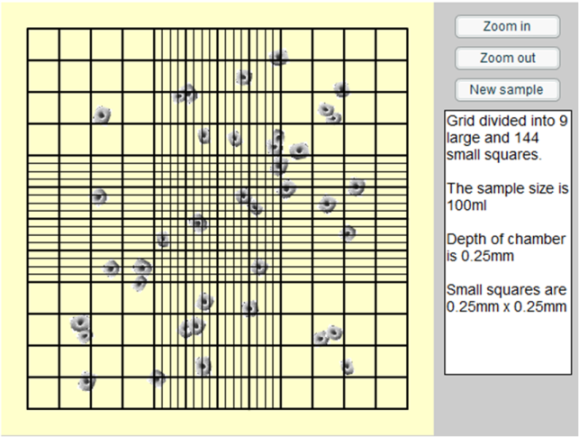You may also likeMake Your Own Solar System

Making a scale model of the solar system

Using the Haemocytometer

Age 14 to 16 Challenge Level:

This problem illustrates calculations typical of those routinely encountered at University-level. Rather than memorising the steps explained below, it is far more beneficial to understand the process by which the answer is obtained.

The problem can be tackled in a few easy steps, which are applicable regardless of the set-up of the Haemocytometer:

1) Count the number of cells you can see - although this seems like an easy task, it should be noted that in many configurations of the haemocytometer it may be difficult to distinguish between overlapping cells. This is particularly illustrative of real laboratory specimens, where cells will often significantly overlap in dense mediums.

2) Work out the volume of a the haemocytometer - this is calculated by multiplying together the length, width and depth of the given square-size (either large or small) and then multiplying this up to give the total volume. The volume of a small square should be multiplied by 144, and the volume of a large square by 9 to give the total volume of the haemocytometer.

It is also important to note that since 1ml corresponds to 1 cm$^3$, converting the length, width and depth measurements into cm from mm will greatly ease the calculation.

3) Work out the number of cells per ml - We have already calculated the number of cells in the haemocytometer, and its volume. Dividing the number of cells by the volume will give the cells per ml (provided that the volume is in cm$^3$)

4) Scale up to the original sample - With the number of cells per ml already calculated, multiplying this number by the appropriate number of ml in the original sample will give the original cell number.

As an example, consider this particular configuration of the Haemocytometer:1) A quick count of the cells reveals that there are 39 present in the haemocytometer.

2) Since the haemocytometer is comprised of 9 large squares, the average number of cells per large square is $\frac{39}{9} = 4.33$

3) Each small square measures 0.25mm x 0.25mm, and so each large square must measure 1mm x 1mm. This is equivalent to 0.1cm x 0.1cm. The depth of a small square is the same as a large square and is given by 0.25mm, which is the same as 0.025cm. Therefore a large square measures 0.1 x 0.1 x 0.025 cm, giving a volume of $2.5 \times 10^{-4} cm^3$, which is the $2.5 \times 10^{-4}$ ml.

4) We have 4.33 cells per large square. Each large square has a volume of $2.5 \times 10^{-4}$ ml. Therefore the number of cells per ml is $\frac{4.33}{2.5 \times 10^{-4}} = 17333$.

5) 17333 cells per ml. Therefore in our sample of 100ml, we have $100 \times 17333 = 1733300 =1.73 \times 10^6$cells.

Can you work out a general formula which allows the calculation of the cells in the sample in a single step?